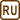## Passing of a train II

### Task number: 387

A passenger train of length $${L_1}=60\,\mathrm{m}$$ travels at speed of $${v_1}=80\,\frac{km}{h}$$ . How long does it take this train to pass a freight train of length $${L_2}=120\,\mathrm{m}$$ traveling at a speed of $${v_2}=30\,\frac{km}{h}$$?

Assume the trains travel:

a) in the same direction.

b) in opposite directions.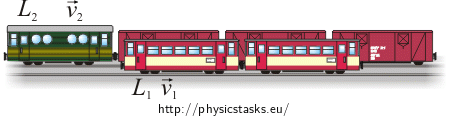• #### Hint 1:

Before you start solving the problem, convert the speed of the trains into base units.

• #### Hint 2:

Draw a picture for both cases.

Imagine that you are the engine driver of the freight train. What is the velocity of the passenger train relative to you in both cases?

• #### Hint 3:

What distance does the passenger train have to travel in order to pass the freight train in both cases?

• #### Hint 4:

You know the distance which the trains have to travel to pass each other. And you also know the speed by which the trains are passing. Therefore you can easily find the time needed by the trains to pass each other.

• #### Solution a) the same direction

First of all we convert the speed of the trains to the base units:

The passenger train: $$v_{1}\,=\,80\,\mathrm{\frac{km}{h}}\,\dot=\, 22.2\,\mathrm{\frac{m}{s}}$$

The freight train: $$v_{2}\,=\,30\,\mathrm{\frac{km}{h}}\,\dot=\, 8.3\,\mathrm{\frac{m}{s}}$$.

We draw a picture of the situation: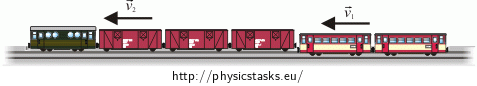When the trains travel in the same direction the speed at which the trains move relative to each other is:

$v_{a}\,=\,v_{1}-v_{2}\,=\,13.9\,\mathrm{\frac{m}{s}}\,.$

The train has to travel the distance which you can see in the picture (the grey passenger train is shown in the place where it starts).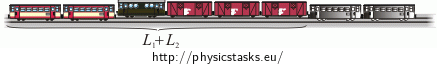The locomotive of the passenger train has to travel the distance:

$L_{1}+L_{2}$

From the determined speed and distance we can now easily find the time needed by the trains to pass each other.

$t_{1}\,=\,\frac{L_{1}+L_{2}}{v_{a}}\,=\,\frac{60 + 120}{13.9}\,\mathrm{s} \,\dot= \,12.9\,\mathrm{s}.$
• #### Solution b) opposite directions

First of all we convert the speed of the trains into base units:

The passenger train: $$v_{1}\,=\,80\,\mathrm{\frac{km}{h}}\,\dot=\, 22.2\,\mathrm{\frac{m}{s}}$$

The freight train: $$v_{2}\,=\,30\,\mathrm{\frac{km}{h}}\,\dot=\, 8.3\,\mathrm{\frac{m}{s}}$$.

We draw a picture of the situation: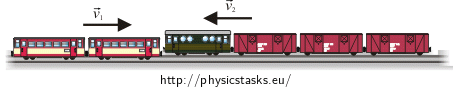When the trains travel in opposite directions the speed by which the trains move relative to each other is:

$v_{b}\,=\,v_{1}+v_{2}\,=\,30.5\,\mathrm{\frac{m}{s}}\,.$

The train has to travel the distance which you can see in the picture (the grey passenger train is shown in the place where it starts).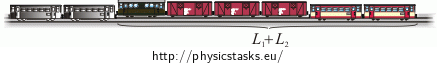The locomotive of the passenger train has to travel the distance:

$L_{1}+L_{2}$

From the determined speed and distance we can easily find the time needed by the trains to pass each other:

$t_{1}\,=\,\frac{L_{1}+L_{2}}{v_{b}}\,=\,\frac{60 + 120}{30.5}\,\mathrm{s} \,\dot= \,5.9\,\mathrm{s}.$
• #### Answer

The passenger train passes the freight train in $$12.9\,\mathrm{s}$$ when the trains travel in the same direction.

When the trains travel in opposite directions, it takes the passenger train $$5.9\,\mathrm{s}$$.

• #### Reference to a similar task

A similar but easier task about a train passing a stationary object is the task Passing of a train I.